This post will guide you how to use Google Sheets MIDB function with syntax and examples.

## 1. Google Sheets MIDB Function Description

The Google Sheets MIDB function returns a substring (a specified number of bytes) starting from the middle of a text string and up to a specified number of bytes.

The MIDB function can be used to extract a given bytes of characters from the middle of a text string in google sheets. It returns a substring from a text string at the position that you specify.

The MIDB function is a build-in function in Google Sheets and it is categorized as a Text Function.

## 2. Google Sheets MIDB Function Syntax

The syntax of the MIDB function is as below:

``= MIDB (text, start_num, length_bytes)``

Where the MIDB function arguments are:

• text -This is a required argument. The text string that you want to extract substring from.
• start_num-This is a required argument.  The position of the first character that you want to extract in text string. The index from the left of text string from which to begin extracting. And the first character in text string is the index 1.
• length_bytes-This is a required argument.  The number of the bytes that you want to extract from a text string.

Note:

• If `start_num`` `is greater than the length of text, the MIDB function will return empty text.
• If `start_num` is less than 1, the MIDB function will return the #VALUE! Error value.
• If `Length_bytesis` negative, MIDB will return the #VALUE!  Error value.
• If you want to extract a substring from start_num to the end of text string, and you can use the LEN function to calculate the length of the text string, you should not specify a large number to `length_bytes` argument for this case.
• If you want to extract a substring beginning with a particular character, and you should use the SEARCH function to get the index of  that character in text string.
• `Length_bytes` must be greater than or equal to 0.
• `Start_num` must be greater than or equal to 1.
• If text string has only single bytes characters and the MIDB function returns the same value as MID function.

## 3. Google Sheets MIDB Function Examples

The below examples will show you how to use google sheets MIDB Text function to extract a substring from a text string.

#1 To extract 10 bytes of substring  from the text string in B1, starting at the 5th character, just using the following formula:

``=MIDB(B1,5,10)  //it returns “le sheets”``

#2 If num_chars value is greater than the length of remaining bytes, and the MID function will return all remining characters:

``=MIDB("google",3,200) //it returns “ogle”``

#3 if text value is a number, and the returned value is also a text by MID function:

``=MIDB(1234,2,2)   //it returns “23”``

Note:

You can use the LEFTB function to extract substring from the left side of a text string in Google Sheets. And you can also use another function called RIGHTB function to extract a substring starting from the right side of the text string. And if you wish to extract a substring starting from the middle position of text string, you can use the MIDB function in google sheets.

## 4. Related Functions

The Google Sheets LEFT function returns a substring (a specified number of the characters) from a text string, starting from the leftmost character.The syntax of the LEFT function is as below:= LEFT(text,[num_chars])…
The Google Sheets LEN function returns the length of a text string (the number of characters in a text string).The syntax of the LEN function is as below:= LEN(text)…

This post will guide you how to use Google Sheets CHAR function with syntax and examples.

## 1. Google Sheets CHAR Function Description

The Google Sheets CHAR function returns the character specified by a number (ASCII Value) according to the current Unicode table. And you can get the Unicode table online at the Unicode website.

The CHAR function can be used to specify a character that are hard to enter in a formula in google sheets. For example, if you wish to enter a line break, and you can use the CHAR(10) function to add a line break to  a text string in a formula in google sheets.

The CHAR function is a build-in function in Google Sheets and it is categorized as a Text Function.

## 2. Google Sheets CHAR Function Syntax

The syntax of the CHAR function is as below:

``=CHAR(number)``

Where the CHAR function argument is:

Number -This is a required argument. the number value is in decimal format and the number of character to look up from the current Unicode table in decimal format.

Note:

• If you provided invalided number, such as: a string value, you will get an error from the Google Sheets CHAR function, it is like to be the “#VALUE!” error
• The CHAR function only have one argument, which must be an valid number in decimal format. The result from Google Sheets CHAR function is a text value. And the CHAR function can be useful when you want to specify a character in formulas or function that are hard to type directly.
• You need to know that not all Unicode characters can be display properly on your computers and you may need to install or enable special fonts or languages so that it can be displayed properly.

## 3. Google Sheets CHAR Function Example

#1 get a character from number 65, 33,  in the computer’s character set

#2 enter one Double Quotes with text in a formula

If you wish to concatenate double quotes with text in Google sheets, and you can use the CHAR function with a number 34 as its argument, type:

=CHAR(34)

## 4. Conclusion

You need know that the CHAR function will only return a single character. If you want to return a string of characters, you can use the CONCATENATE function to combine multiple CHAR functions.

This post will guide you how to use Google Sheets BAHTTEXT function with syntax and examples.

## Description

The Google Sheets BAHTTEXT function Converts a number to Thai text with the suffix Baht for integer values and Satang for decimal values.

The BAHTTEXT function is a build-in function in Google Sheets and it is categorized as a Text Function.

## Syntax

The syntax of the BAHTTEXT function is as below:

= BAHTTEXT(number)

Where the BAHTTEXT function arguments are:

• number -This is a required argument. It is the numeric value that you want to convert into Thai text.

Note:

• If the supplied number argument is not recognized as a numeric value in google sheets, the BAHTTEXT function returns #VALUE! Error message.

## Google Sheets BAHTTEXT Function Examples

The below examples will show you how to use google sheets BAHTTEXT function to convert a number into Thai text with the suffix “Baht”.

#1 To convert a number in Cell B1 to Thai text, just using the following formula:

`= BAHTTEXT(B1)  //it returns “หนึ่งร้อยยี่สิบบาทถ้วน”`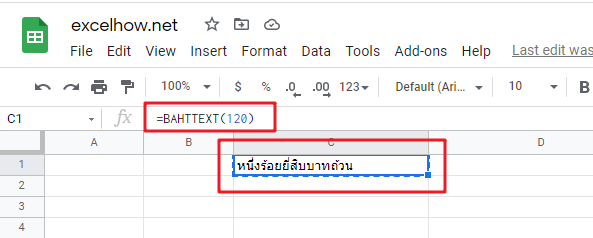This post will guide you how to use Google Sheets TEXTJOIN function with syntax and examples.

## Description

The Google Sheets TEXTJOIN function joins two or more text strings together and separated by a delimiter. you can select an entire range of cell references to be combined in google sheets. and you can also specify an empty string as delimiter to include between each text in different cells.

The TEXTJOIN function can be used to combine the text from multiple text strings or arrays with a specifiable delimiter in google sheets. The purpose of this function is to join text values with a given delimiter and its returned values is a concatenated text.

The TEXTJOIN function is a build-in function in Google Sheets and it is categorized as a Text Function.

## Syntax

The syntax of the TEXTJOIN function is as below:

=TEXTJOIN (delimiter, ignore_empty,text1,[text2])

Where the TEXTJOIN function arguments are:

• delimiter -This is a required argument. It can be a text string or empty string to join text values with a given delimiter, it can be a space, comma, hash or other text string.
• Ignore_empty – This is a required argument.  If TRUE, empty cells or string value should be ignored.
• Text1/Text2 – This is a required argument.  One or more strings that you want to join together.

Note:

• the text arguments should not exceed 252 and the length of resulting string should not exceed the cell limit of 32767.
• If you want to concatenate text strings manually, and you can use the concatenation operator(&)
• The CONCATENATE function only provides basic concatenation
• If a number is provided to TEXTJOIN function, and it will convert it as a text values during concatenation.

## Google Sheets TEXTJOIN Function Examples

The below examples will show you how to use google sheets TEXTJOIN function to concatenate the items of one or more text strings using a specified delimiter.

#1 To join strings in B1,C1,D1 cells, just using formula:

`= TEXTJOIN (",",TRUE,B1,C1,D1)  //it returns “google,sheets,learning”`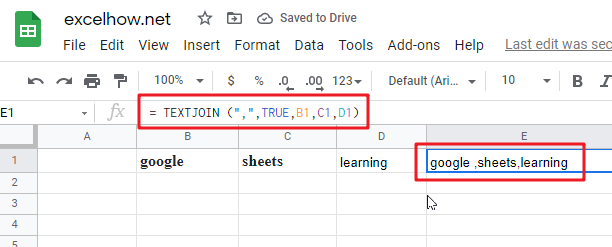#2 while you want to concatenate the strings by specifying multiple delimiter and order, you can use the following formula:

`=TEXTJOIN({"@","."},TRUE,B1:D1) //it returns “google@sheets@com”`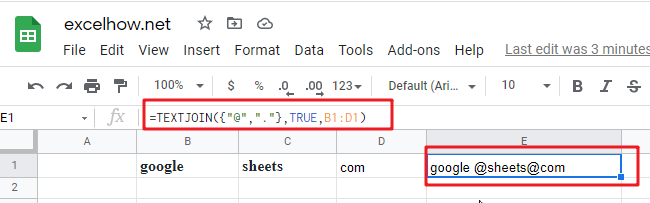#3 To join values from the multiple cell ranges with a double dash character as delimiter. using the following formula:

`=TEXTJOIN("–",TRUE,B1:B6,C1:C6) //it returns “g-o-o-g-l-e-s-h-e-e-t-s”`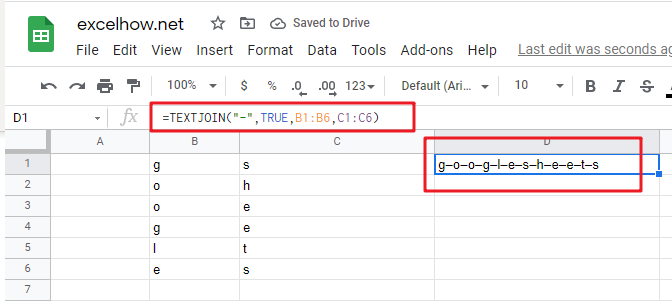#4 to join string in range cell A1:C1 by a comma character as delimiter and empty cells should not be ignored. using the following formula:

`=TEXTJOIN(",",FALSE,A1:C1) //it returns “google,,sheets”`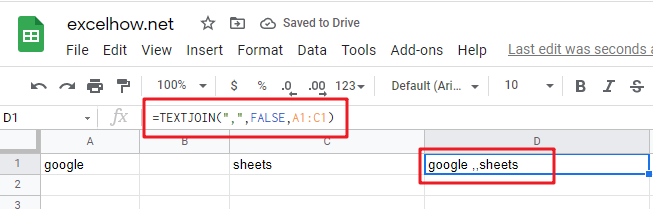This post will guide you how to use Google Sheets VALUE function with syntax and examples.

## Description

The Google Sheets VALUE function converts a text string that represents a number to a numeric value.

The VALUE function can be used to converts a text string in the date, time or number formats into a numeric value in google sheet. The purpose of this function is to convert text to a number and its returned values is a numeric value.

The VALUE function is a build-in function in Google Sheets and it is categorized as a Text Function.

## Syntax

The syntax of the VALUE function is as below:

= VALUE (text)

Where the VALUE function argument is:

• Text -This is a required argument. The text string that you want to convert it to a number.

Note:

• Text can be in any of the constant number, date, or time formats. And if text is not a number, the VALUE function will return #VALUE! Error.
• If the VALUE function is not able to convert a text value to a numeric result, it returns a #VALUE! Error message.
• VALUE function is provided for compatibility with other spreadsheet applications.

## Google Sheets VALUE Function Examples

The below examples will show you how to use google sheets VALUE function to convert string to a number.

#1 To convert a text in B3 cell to a number, just using formula:

`= VALUE(B3)`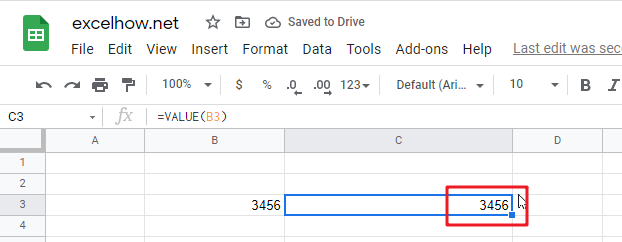#2 to convert a text string that is not a recognized format (number, date, time format) into a numeric value, using the below formula:

`=VALUE(B5)`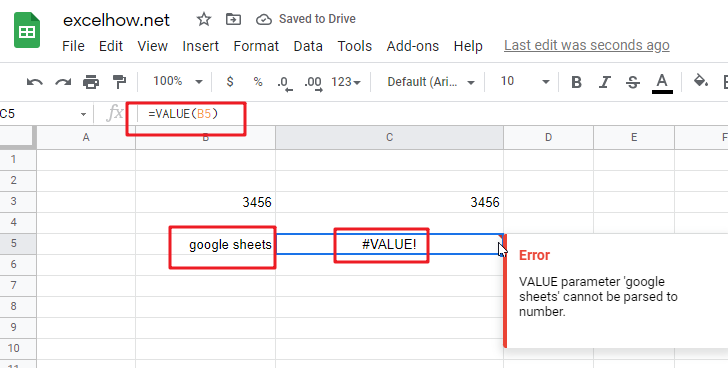You will see that the #VALUE! Error will be returned by the VALUE function. Because the text is not a number.

This post will guide you how to use Google Sheets UPPER function with syntax and examples.

## Description

The Google Sheets UPPER function converts all characters in text string to uppercase.

The UPPER function can be used to convert a given text string to uppercase letters in google sheet. And you need to know that numbers, spaces and punctuation are not affected in this function.

The UPPER function is a build-in function in Google Sheets and it is categorized as a Text Function.

## Syntax

The syntax of the UPPER function is as below:

= UPPER(text)

Where the UPPER function arguments are:

• text -This is a required argument. The text string that you want to convert to uppercase.

Note:

• The UPPER function only takes one argument. It converts a text string to uppercase letters. And one argument can be a text string or a cell reference.
• Numbers characters inside a text string are not affected in UPPER function.

## Google Sheets UPPER Function Examples

The below examples will show you how to use google sheets UPPER Text function to convert all lowercase characters in a text string to uppercase.

#1 To upper case all characters in B1 cell, just using the following formula:

`=UPPER(B1) //it returns GOOGLE SHEET`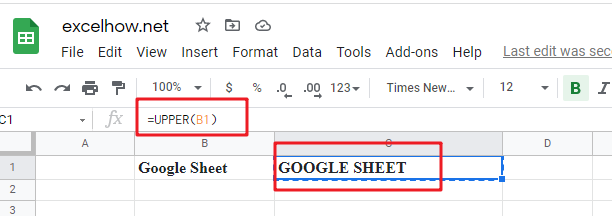#2 Using UPPER function to convert a text string containing numbers or punctuation characters, just using formula:

`=UPPER("gOogle sheet-2021")  //it returns “GOOGLE SHEET-2021”`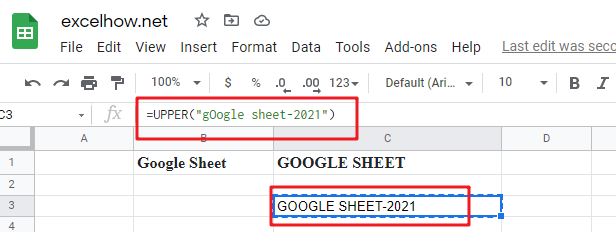Note:

You can use the LOWER function to convert a specified string to lowercase in Google Sheets. And you can also use another function called UPPER function to convert a specified string to uppercase. And if you wish to capitalize each wrd in a specified string, you can use the PROPER function in google sheets.

This post will guide you how to use Google Sheets UNICODE function with syntax and examples.

## Description

The Google Sheets UNICODE function returns the decimal Unicode value of the first character of the text.

The UNICODE function can be used to return a number corresponding to a Unicode character in google sheets.  The purpose of this function is to get number from a Unicode character and its returned value is a decimal number.

The UNICODE function is a build-in function in Google Sheets and it is categorized as a Text Function.

## Syntax

The syntax of the UNICODE function is as below:

= UNICODE(text)

Where the UNICODE function argument is:

• text -This is a required argument. The string containing the character to be converted

Note:

• Unicode can be implemented by different character encodings. The Unicode standard defines Unicode Transformation Formats (UTF): UTF-8, UTF-16, and UTF-32, and several other encodings.
• The UNICODE function only words properly if the input text string is not empty and the first character has a Unicode representation.
• Number can be passed directly into the UNICODE function, returning as a string.

## Google Sheets UNICODE Function Examples

The below examples will show you how to use google sheets UNICODE function to convert a Unicode character to a number.

#1 To get a number from a Unicode character in cell B1, just using the following formula:

`=UNICODE(B1)  //it returns E`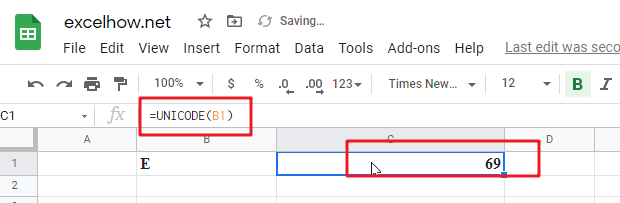Note: you can use the UNICHAR function to perform the opposite conversion to return the Unicode character for a number.

This post will guide you how to use Google Sheets UNICHAR function with syntax and examples.

## Description

The Google Sheets UNICHAR function returns the Unicode character for a given number. This function support to return characters in both the UTF-8 and UTF-16 character set.

The UNICHAR function can be used to returns a Unicode character based on a given number in google sheets.  The purpose of this function is to get Unicode character by a number and its returned value is a Unicode character.

The UNICHAR function is a build-in function in Google Sheets and it is categorized as a Text Function.

## Syntax

The syntax of the UNICHAR function is as below:

= UNICHAR(number)

Where the UNICHAR function argument is:

• number -This is a required argument. The number to convert into a Unicode character.

Note:

• Unicode is a character set. It is a very large character set. And it contains 137439 characters.
• If number argument is 0, the UNICHAR function returns a #VALUE! Error message.
• If the number does not have a corresponding Unicode character, and the UNICHAR function returns a #VALUE! Error.

## Google Sheets UNICHAR Function Examples

The below examples will show you how to use google sheets UNICHAR function to convert a number to Unicode character.

#1 To get a Unicode character for a number value in cell B1, just using the following formula:

`=UNICHAR(B1)  //it returns E`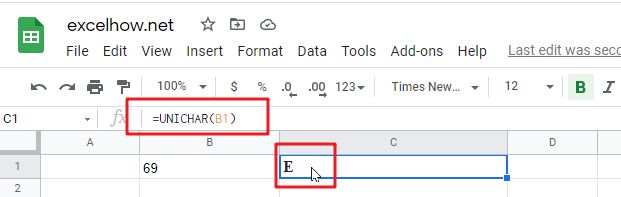This post will guide you how to use Google Sheets TRIM function with syntax and examples.

## Description

The Google Sheets TRIM function removes all spaces from text string except for single spaces between words.  You can use the TRIM function to remove extra spaces between words in a string. Or removes leading and trailing spaces from the text.

The TRIM function can be used to remove extra spaces from a given text string, it will leave only a single space between words in google sheets.  The purpose of this function is to remove extra spaces from text. and its returned value is a text with extra spaces removed.

The TRIM function is a build-in function in Google Sheets and it is categorized as a Text Function.

## Syntax

The syntax of the TRIM function is as below:

= TRIM (text)

Where the TRIM function argument is:

• Text -This is a required argument. The text string from which you want to remove the extra spaces.

Note:

• TRIM Function only removes extra spaces in a text string, leaving just a single space between words.
• TRIM function is very important when text is used in formulas or data validation.
• TRIM function only removes the ASCII space character from a text string.
• Whitespace or non-breaking space will not be trimmed. If you

## Google Sheets TRIM Function Examples

The below examples will show you how to use google sheets TRIM function to remove extra spaces.

#1 To remove leading spaces in the Cell B1, Just using the following formula:

`=TRIM(B1)`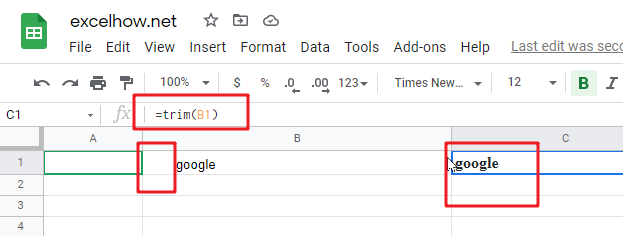#2 To remove multiple spaces between two words in B2, using the below excel formula:

`=TRIM(B2)`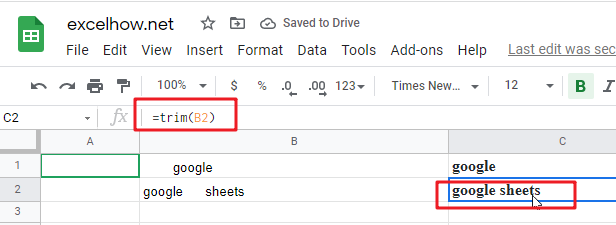This post will guide you how to use Google Sheets TEXT function with syntax and examples.

## Description

The Google Sheets TEXT function converts a numeric value into text string with a specified format.

The TEXT function can be used to convert a number formatted as text according to a specified format in google sheets.  The purpose of this function is to convert a number to text in a number format. and its returned value is a number as text in the given format.

The TEXT function is a build-in function in Google Sheets and it is categorized as a Text Function.

## Syntax

The syntax of the TEXT function is as below:

= TEXT (value, Format code)

Where the TEXT function arguments are:

• number -This is a required argument. The value you want to format.
• Format code– This is a required argument. The format code that you want to apply.

The “`Format Code`” can be used in the excel Text function are shown in the below table.

 Format Code Description Examples 0 only display digits in its place #.00 – Forces the function to display two decimal places =Text(34.234,”\$##.00″) result: \$34.23 # Display the placeholder =Text(4.527,”#.##) result: 4.53 . the position of Decimal Point =Text(342.2,”0.00″) result: 342.20 d Day of the month or day of week d- one or two digit number (1-31) dd- two digit number (01-31) ddd-abbreviated day of week (Mon to Sun) dddd-full name of day of week(Monday to Sunnday) =Text(TODAY(),”DDDD”) result: Monday m The Month of the Year m- one or two digit number mm-two digit number mmm-abbreviated month(Jan to Dec) mmmm-full name of month(January to December)) =Text(TODAY(),”MM/DD/YY”) result:11/06/17 y year yy-two digit representation of year(e.g.01,17) yyyy-four digit representation of year(e.g. 2001,2017) =Text(TODAY(),”MM/DD/YY”) result:11/06/17 h Hour h-one or two digit number (e.g. 1,23) hh-two digit number (e.g. 01,23) =Text(14:16,”hh:mm”) result: 14:16 m Minute m-one or two digit representation (e.g. 1,59) mm-two digit representation (e.g. 01,59) =Text(14:16,”hh:mm”) result: 14:16 s Second s-one or two digit representation (e.g. 1,59) ss=two digit representation (e.g. 01,59)

Important Notes:

• The Text formula converts a numeric value to formatted text, but its result can not be used for calculation purpose in the other excel functions or formula.
• the “format code must be marked in the quotation marks. or it will return “#NAME?” error.
• the asterisk character cannot be used in format code.
• The TEXT function takes two arguments, `value` and `format_code`. `Value` is the number, date or time to format. and it should be a numeric value. `Format_code` is a pattern by which to format the number.
• The output of TEXT function is always a text string. If you want to format a number, just apply a regular number formatting in google sheets.

## Google Sheets TEXT Function Examples

The below examples will show you how to use google sheets TEXT function to convert a numeric value into a text string with specified format code.

#1 To convert a numeric value(34293) with MM/DD/YY format code in B1 cell, just using formula:

`= TEXT(B1,"MM/DD/YY") //it returns 11/20/93`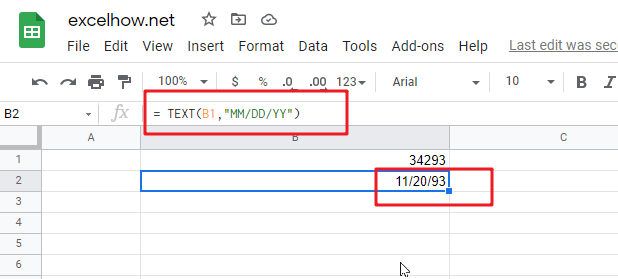This post will guide you how to use Google Sheets T function with syntax and examples.

## Description

The Google Sheets T function returns the text referred to by value. You can use T function to remove values that are not text.

The T function can be used to return text when given a text value as numbers, dates or the logic values in google sheets.  The purpose of this function is to filter text values only. And its returned output is also a text.

The T function is a build-in function in Google Sheets and it is categorized as a Text Function.

## Syntax

The syntax of the T function is as below:

= T (value)

Where the T function argument is:

• value -This is a required argument. The value you want to be converted text.

Note:

• The T function only takes one argument, `value`, which can be a cell reference, or a text value.
• If the `value` does not refer to a text string, the T function will return an empty text.
• If `value` is text, T function returns `value`.
• If `value` is a cell reference that containing text value, T function returns the content of `value`.
• If `value` is an error or a cell that containing an error, T function returns the error.

## Google Sheets T Function Examples

The below examples will show you how to use google sheets T function to return the text string referred to by value.

#1 To return the text string value in B1, just using formula:

`=T(B1) // it returns “learn google sheets free - google function”`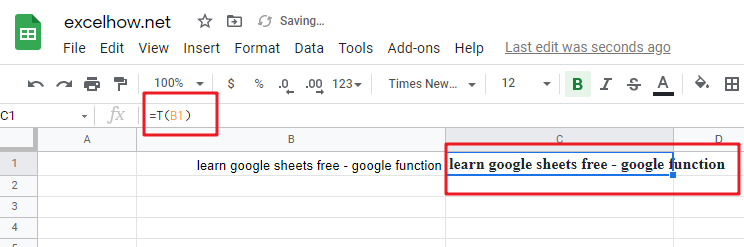#2 to return the text string value in cell B1 that containing a numreic value, using the following T function:

`=T(B1)  //it returns “”`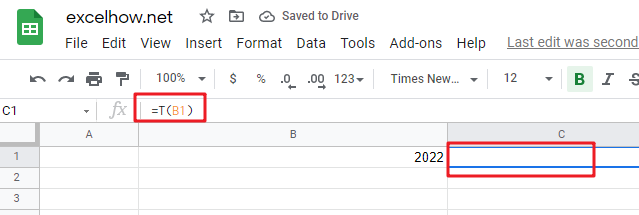Note: The T function is unnecessary. As Google sheets will convert between most formats automatically. And it is provided for compatibility with formulas used in other spreadsheet programs.

This post will guide you how to use Google Sheets SUBSTITUTE function with syntax and examples.

## Description

The Google Sheets SUBSTITUTE function replaces a new text string for an old text string in a text string.

The SUBSTITUTE function can be used to replace existing text with new text in a string in google sheets.  The purpose of this function is to replace text based on content. And its returned value is the processed text.

The SUBSTITUTE function is a build-in function in Google Sheets and it is categorized as a Text Function.

## Syntax

The syntax of the SUBSTITUTE function is as below:

=SUBSTITUTE(text, old_text, new_text,[instance_num])

Where the SUBSTITUTE function arguments are:

• Text -This is a required argument. The text string that containing the old text to be substituted with new text string.
• Old_text – This is a required argument. The substring that you want to replace.
• New_text – This is a required argument. The new substring to replace Old_text substring with.
• Instance_num– This is an optional argument.  The number value will specify which occurrence of old substring you want to replace with new substring. By default, all occurrences of old_text are replaced.

Note:

• If Instance_numvalue is omitted, every occurrence of old substring will be replaced with new substring.
• The SUBSTITUTE function used to replace text based on matching and not position.
• The SUBSTITUTE function is case-sensitive.
• Old_text will match parts of words as well as whole words. For example, searching a string “sh” will also replace text within “sheets”.
• SUBSTITUTE function can only be used to replace one or all instances of a string within `new_text` in a single cell.
• SUBSTITUTE function returns text as the output. If a number output is desired, and you can try using the VALUE function in combination with this function.

## Google Sheets SUBSTITUTE Function Examples

The below examples will show you how to use google sheets SUBSTITUTE function to replace old substring with a new substring.

#1 To substitute first instance of “google”old text string with a new text string “mygoogle” in B1 cell, just using formula:

`=SUBSTITUTE(B1,”google”,” mygoogle”,1).`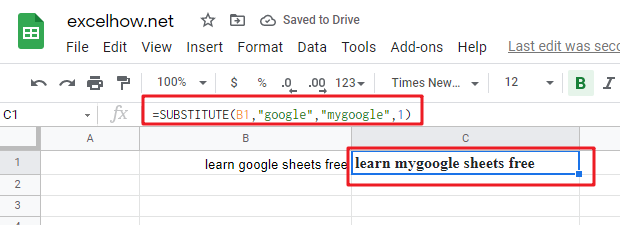#2 To substitute second instance of “google”old text string with a new text string “googlefunction” in B1 cell, just using formula:

`=SUBSTITUTE(B1,”google”,”googlefunction “,2)`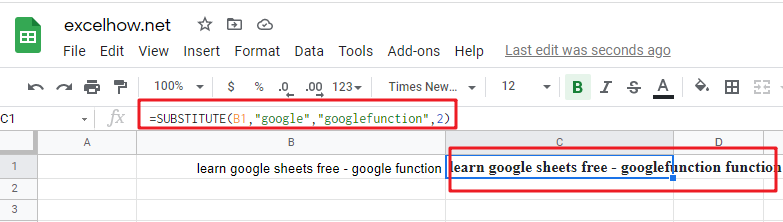Note: You can use the REPLACE function to replace a newly text string at a given position in a text string. And you can use the SUBSTITUTE function to replace an existing text with a new text in a string. And Using FIND function or SEARCH function to get the location of a given text string in a text sting.

This post will guide you how to use Google Sheets SPLIT function with syntax and examples.

## Description

The Google Sheets SPLIT function used to divide text string around a specified character or delimiter and put each separate pieces into a separate cell in the row.

The SPLIT function can be used to divide a text string or text value around a given delimiter in google sheets.  The purpose of this function is to split a text string based on a delimiter.

The SPLIT function is a build-in function in Google Sheets and it is categorized as a Text Function.

## Syntax

The syntax of the SPLIT function is as below:

=SPLIT(text, delimiter, [split_by_each], [remove_empty_text])

Where the SPLIT function arguments are:

• text -This is a required argument. The text string or value that you want to split. It can also be a cell reference or a cell or the output of a nested formula. it should be a string and not an array result.
• delimiter -This is a required argument. The delimiter is the character or characters that used to split the text. By default, each character in delimiter is considered individually.  For example, if delimiter is “test”, then text string is divided around the characters “t”,”h” and “e”.  You can set split_by_each argument to FALSE to disable this behavior.
• split_by_each -This is an optional argument. This argument will control whether or not to divide text around each character contained in delimiter argument. The default value is TRUE. And the TRUE behavior will split text by individual characters in the delimiter. And The FALSE behavior only divides text by the entire delimiter.
• remove_empty_text – This is an optional argument. This argument will control whether or not to remove empty text messages from the split results. The default value is TRUE.

Note:

• Delimiters are case sensitive. So “lowercase “g” is only splits on lower-cases g’s in the text string.
• The SPLIT function requires enough cells for its output. If it splits a text string into 3 elements, and it requires 3 empty cells on the same row to place them. If there is already data in those cells, it does not overwrite those cells, then it shows a #REF! error message.

## Google Sheets SPLIT Function Examples

The below examples will show you how to use google sheets SPLIT function to split a text string around a given delimiter.

#1  To separate a list of names in Cell B1 with “,” delimiter character, just using the following formula:

`=SPLIT(B1,”,”)`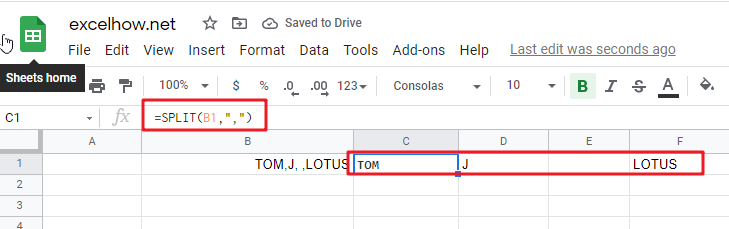The above SPLIT formula will separate names using the comma character as a delimiter. And the result is 4 cells, and each cell contain a name.  and you can notice that there is one blank cell, because the text string in Cell B1 has two adjacent commas with a space character between those two commas.

This post will guide you how to use Google Sheets SEARCHB function with syntax and examples.

## Description

The Google Sheets SEARCHB function returns the number of the starting location of a substring in a text string counting each double-character as 2. You can use the SEARCHB function to locate a text string within another text string and returns the position of the first character of find_text within text.

The SEARCHB function can be used to calculate the position at which a string is first found within text counting each double-character as 2 in google sheets.  The purpose of this function is to get the location of text in the given string. And it’s returned value as a number representing the location of finding text.

The SEARCHB function is a build-in function in Google Sheets and it is categorized as a Text Function.

## Syntax

The syntax of the SEARCHB function is as below:

= SEARCHB(find_text, within_text,[start_num])

Where the SEARCHB function arguments are:

• find_text -This is a required argument. The substring that you want to find
• within_text -This is a required argument. The text string in which you want to search for the substring (find_text).
• Start_num -This is an optional argument. The starting position to search.

Note:

• The SEARCHB function will return the position of a find_text inside another text string. And if there is more than one occurrence of the find string, and the SEARCHB function returns the position of the first occurrence.
• If `find_text` string is not found, the SEARCHB function will return the #VALUE! Error value.
• When find text string is empty, the SEARCHB function will return 1.
• If `start_num` value is omitted, it is set to be 1 by default.
• The SEARCHB function is not case-sensitive. For example, “google” will match “GOOGLE”. If you wish to compare text where case matters, and you can use the FIND function.
• The SEARCHB function support wildcard characters.
• you can use another function called IFERROR function in combination with the SEARCHB function to check for cases when there are any matches to the search.
• Using SEARCH function for standard character sets and SEARCHB function for double-byte character sets such as Chinese, Japanese, and Korean.

## Google Sheets SEARCHB Function Examples

The below examples will show you how to use google sheets SEARCHB function to get the position of substring in a text string.

#1 To get the position of “google” text string in B1 cell, starting at the second position, just using formula:

`= SEARCHB(“google”,B1,2) //it returns 7`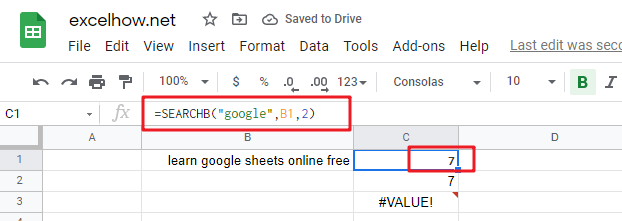#2 The search function use wildcard characters to look for a string starts with “go” characters in cell B1, using the following formula:

`=SEARCHB(“go*”,B1,1) //it returns 7`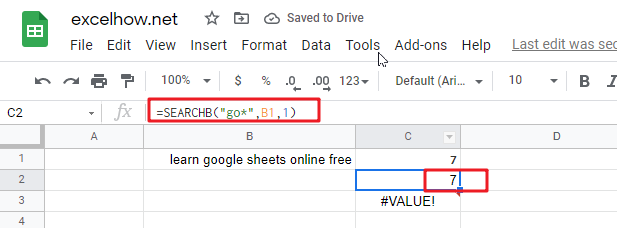#3 #VALUE! Error will be returned if find_text string is not found in with_text:

`=SEARCH(“test”,”google”,1)   //it returns #VALUE!`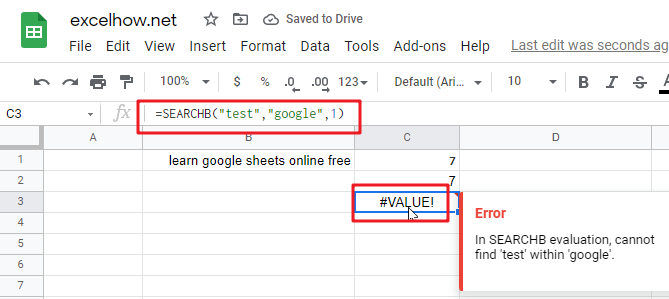#4 to get the position of a double-byte character sets “新“ within another Chinese text string, using the following formula:

`=SEARCHB("新", "农历新年", 2) //it returns 5`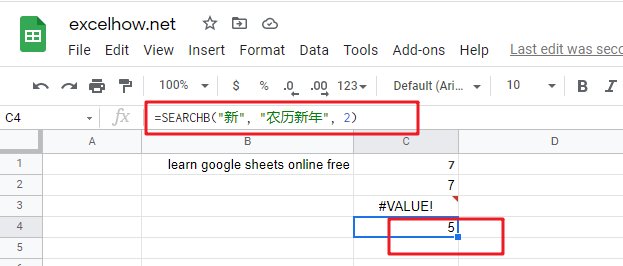This post will guide you how to use Google Sheets SEARCH function with syntax and examples.

## Description

The Google Sheets SEARCH function returns the number of the starting location of a substring in a text string. You can use the SEARCH function to locate a text string within another text string and returns the position of the first character of find_text within text.

The SEARCH function can be used to calculate the position at which a string is first found within text in google sheets.  The purpose of this function is to get the location of text in the given string. And it’s returned value as a number representing the location of finding text.

The SEARCH function is a build-in function in Google Sheets and it is categorized as a Text Function.

## Syntax

The syntax of the SEARCH function is as below:

= SEARCH (find_text, within_text,[start_num])

Where the SEARCH function arguments are:

• find_text -This is a required argument. The substring that you want to find
• within_text -This is a required argument. The text string in which you want to search for the substring (find_text).
• Start_num -This is an optional argument. The starting position to search.

Note:

• The SEARCH function will return the position of a `find_text` inside another text string. And if there is more than one occurrence of the find string, and the SEARCH function returns the position of the first occurrence.
• If `find_text` string is not found, the search function will return the #VALUE! Error value.
• When find text string is empty, the SEARCH function will return 1.
• If `start_num` value is omitted, it is set to be 1 by default.
• The SEARCH function is not case-sensitive. For example, “google” will match “GOOGLE”. If you wish to compare text where case matters, and you can use the FIND function.
• The SEARCH function support wildcard characters.
• you can use another function called IFERROR function in combination with the SEARCH function to check for cases when there are any matches to the search.

## Google Sheets SEARCH Function Examples

The below examples will show you how to use google sheets SEARCH function to get the position of substring in a text string.

#1 To get the position of “google” text string in B1 cell, starting at the second position, just using formula:

`=SEARCH(“google”,B1,2) //it returns 7`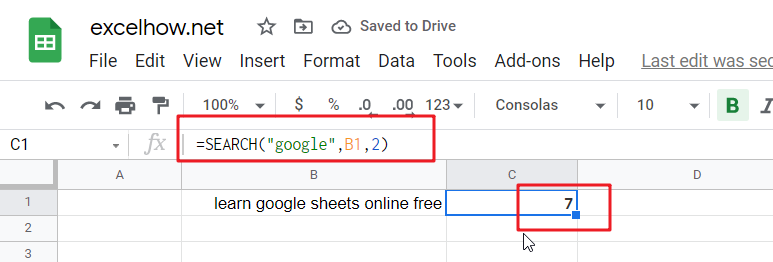#2 The search function use wildcard characters to look for a string starts with “go” characters in cell B1, using the following formula:

`=SEARCH(“go*”,B1,1) //it returns 7`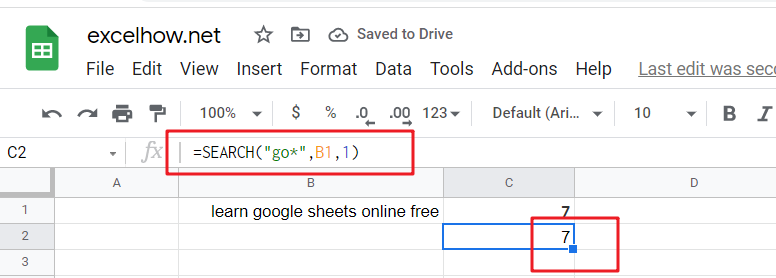#3 #VALUE! Error will be returned if find_text string is not found in with_text:

`=SEARCH(“test”,”google”,1)   //it returns #VALUE!`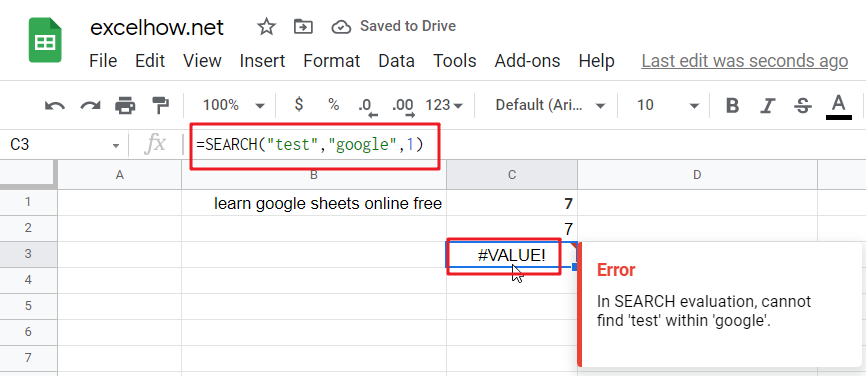This post will guide you how to use Google Sheets ROMAN function with syntax and examples.

## Description

The Google Sheets ROMAN function converts a given number to a Roman numeral as text result.

The ROMAN function can be used to format a number in Roman numerals in google sheets. And it’s returned value as a roman numeral in text. And it’s purpose is to convert numbers to Roman numerals.

The ROMAN function is a build-in function in Google Sheets and it is categorized as a Text Function.

## Syntax

The syntax of the ROMAN function is as below:

= ROMAN (number, [rule_relaxation])

Where the ROMAN function arguments are:

• Text -This is a required argument. The number to format, between 1 and 3999.
• rule_relaxation -This is an optional argument. The type of Roman numeral that you want to convert. The default form is 0.

Note:

• ROMAN function takes two arguments, `number` and `rule_relaxation`. The first argument, `number` should be a whole number between 0-3999. And the second argument, `rule_relaxation` is the degree to which traditional syntax rules may be relaxed. And the default value is zero. And other values are 1-4.
• Number should be a positive number between 1 and 3999.
• If number argument is a negative value or out of range (0-3999), and the ROMAN function returns #VALUE! Error message.

## Google Sheets ROMAN Function Examples

The below examples will show you how to use google sheets RIGHTB function to extract the rightmost substring from a text string.

#1 Convert a number 1000 to a Roman numeral, using the following formula:

`=ROMAN(1000)  //it returns “CDXCIX”`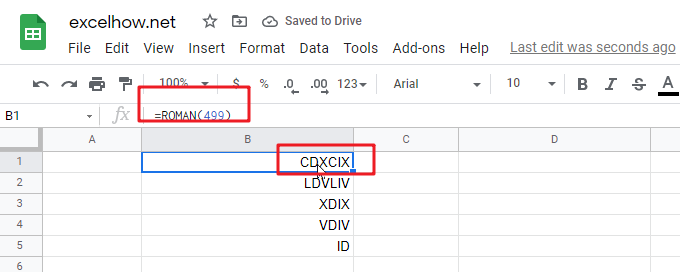#2 Convert a number 1000 to a Roman numeral with different rule relaxation, using the following formulas:

```=ROMAN(1000,1)  //it returns “LDVLIV”
=ROMAN(1000,2)  //it returns “XDIX”
=ROMAN(1000,3)  //it returns “VDIV”
=ROMAN(1000,4)  //it returns “ID”’’```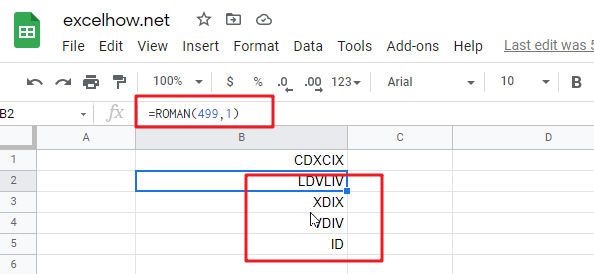Note: if you want to convert Arabic numbers to Roman number, you can use the ROMAM function. And if you wish convert Roman numbers to Arabic numbers, you can use the ARABIC function in google sheets.

This post will guide you how to use Google Sheets RIGHTB function with syntax and examples.

## Description

The Google Sheets RIGHTB function returns a substring (a specified number of the bytes) from a text string, starting from the right portion.

The RIGHTB function can be used to extract the right portion of a string up to a certain number of bytes in google sheets.

The RIGHTB function is a build-in function in Google Sheets and it is categorized as a Text Function.

## Syntax

The syntax of the RIGHTB function is as below:

= RIGHTB(text,[num_of_bytes])

Where the RIGHTB function arguments are:

• Text -This is a required argument. The text string that you want to extract from.
• num_of_bytes -This is an optional argument. It will specify the number of bytes that you want to extract from Text string.

Note:

• The `num_of_bytes` value must be greater than or equal to zero. If `num_of_bytes`value is greater that the length of text string, it will return all of text string. If `num_of_bytes `value is omitted, it is set to be 1 by default.
• If `Num_bytes` equal to 0, it will cause RIGHTB function to extract the empty string.
• If text string has only single bytes characters and the RIGHTB function returns the same value as RIGHTB function.

## Google Sheets RIGHTB Function Examples

The below examples will show you how to use google sheets RIGHTB function to extract the rightmost substring from a text string.

#1 To extract the rightmost 4 bytes in the B1 cell, just using formula:

`= RIGHTB(B1,4) //it returns “ogle”`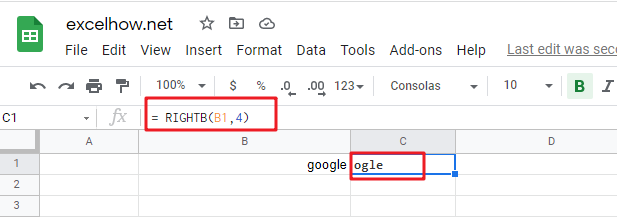#2 If you do not provide num_of_bytes argument, it will use the default value as 1, using the following formula:

`= RIGHTB ("google") //it returns “e”`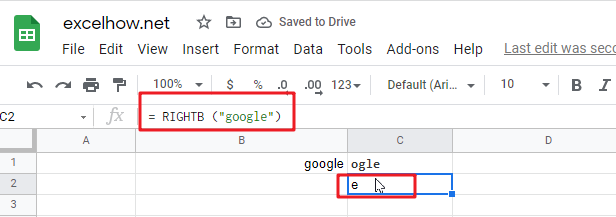#3 If Num_chars exceeds the text string length, the RIGHTB function will return the entire string:

`= RIGHTB ("google",200)  //it returns “google”`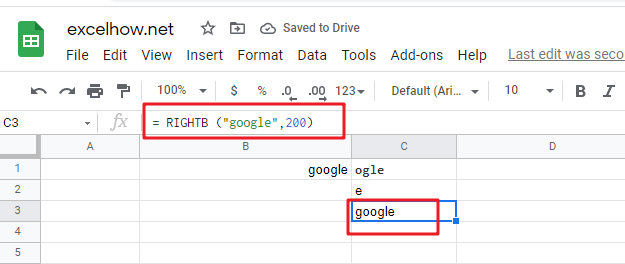#4 If Text value is a numeric value, and the RIGHTB function will return a text value:

`= RIGHTB (1234,2) //it returns “34”`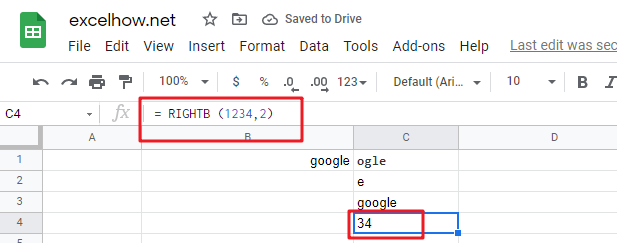Note:

You can use the LEFTB function to extract substring from the left side of a text string in Google Sheets. And you can also use another function called RIGHTB function to extract a substring starting from the right side of the text string. And if you wish to extract a substring starting from the middle position of text string, you can use the MIDB function.

This post will guide you how to use Google Sheets RIGHT function with syntax and examples.

## Description

The Google Sheets RIGHT function returns a substring (a specified number of the characters) from a text string, starting from the rightmost character.

The RIGHT function can be used to extract a given number of characters from the rightmost of a text string in google sheets. It returns a substring from the beginning of a specified string.  It’s purpose is to extract text from the right of a text string.

The RIGHT function is a build-in function in Google Sheets and it is categorized as a Text Function.

## Syntax

The syntax of the RIGHT function is as below:

=RIGHT(text,[num_chars])

Where the RIGHT function arguments are:

• Text -This is a required argument. The text string that you want to extract from.
• num_chars -This is an optional argument. It will specify the number of characters that you want to extract from Text string.

Note:

• The `Num_chars` value must be greater than or equal to zero. If `Num_chars` is greater that the length of text string, it will return all of text string. If `Num_chars` value is omitted, it is set to be 1 by default.
• If `Num_chars` is equal to 0, it will cause RIGHT function to extract the empty string.
• The RIGHT function takes two arguments, `text` and `num_chars`. The first argument, `text` is the string from which the right portion will be returned. And the second argument, `num_chars` is the number of characters to return from the right side of text.

## Google Sheets RIGHT Function Examples

The below examples will show you how to use google sheets RIGHT Text function to extract the rightmost substring from a text string.

#1 To extract the rightmost 4 characters in the B1 cell, just using formula:

`=RIGHT(B1,4) //it returns “ogle”`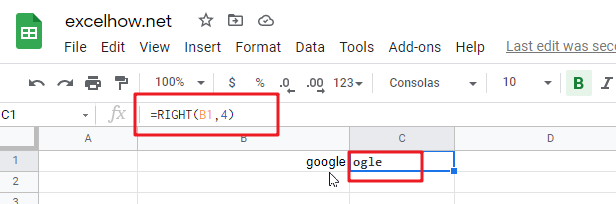#2 If you do not provide num_chars argument, it will use the default value as 1, using the following formula:

`=RIGHT(“google”) //it returns “e”`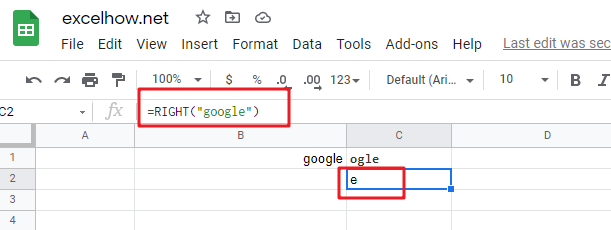#3 If Num_chars value exceeds the text string length, the RIGHT function will return the entire string:

`=RIGHT("google",200)  //it returns “google”`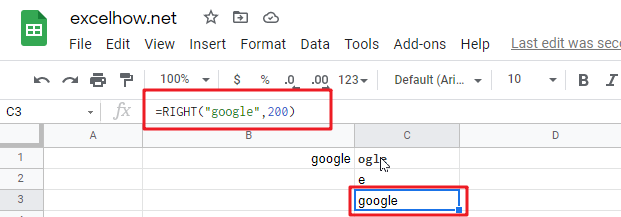#4 If Text value is a numeric value, and the RIGHT function will return a text value:

`= RIGHT(1234,2) //it returns “34”`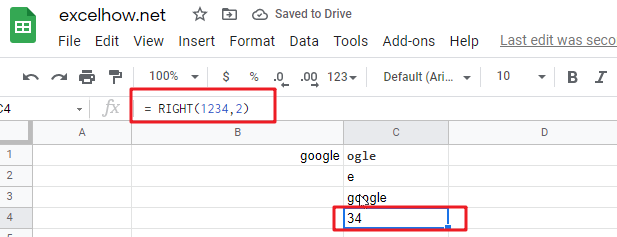#5 You can also use the RIGHT function in combination with the FIND function to create a complex formula to extract a specific text string. The FIND function will get the position of the given charcter in text string, and the RIGHT function returns all text characters to the right of that position in google sheets.

`= RIGHT ("google",FIND("l","google")-1)  //it returns “ogle”`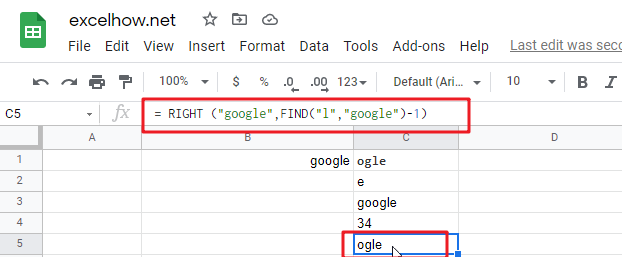Note:

You can use the LEFT function to extract substring from the left side of a text string in Google Sheets. And you can also use another function called RIGHT function to extract a substring starting from the right side of the text string. And if you wish to extract a substring starting from the middle position of text string, you can use the MID function.

## Related Functions

The Google Sheets FIND function returns the position of the first text string (sub string) within another text string.The syntax of the FIND function is as below:= FIND(find_text, within_text,[start_num]) …
The Google Sheets MID function returns a substring (a specified number of the characters) starting from the middle of a text string. The syntax of the MID function is as below:= MID (text, start_num, num_chars)…

This post will guide you how to use Google Sheets REPT function with syntax and examples.

## Description

The Google Sheets REPT function repeats a text string a specified number of times.

The REPT function can be used to repeat specified text repeated a number of times in google sheets. And it’s return value as the repeated text. The purpose of this function is repeat text as specified.

The REPT function is a build-in function in Google Sheets and it is categorized as a Text Function.

## Syntax

The syntax of the REPT function is as below:

= REPT (text, number_times)

Where the REPT function arguments are:

• Text -This is a required argument. The text string that you want to repeat.
• Number_times – This is a required argument.  The number of times that you want to repeat

Note:

• If number_times is zero, the REPT function will return empty text. And If `number_times` value is not an integer, it is truncated to an integer value.
• `Nuber_times` argument should be zero or a positive integer, otherwise, it will return an #VALUE error.
• REPT function takes two arguments, `text` and `number_times`. The first argument, `Text` is the characters to repeat. And the second argument, `number_times` is the number of times text should appear in the value returned.
• REPT function does not insert any space characters between repetitions of text. If you wish to insert a space, a space character must be appended to the end of the value of text value. And the resulting return value from REPT function will have at least one trailing space, and you can use another function called TRIM function to remove it.

## Google Sheets REPT Function Examples

The below examples will show you how to use google sheets REPT function to repeat a text string a given number of times.

#1 To repeat a text string in B1 cell 3 times, just using the following formula:

`=REPT (B1,3) //it returns “googlegooglegoogle”`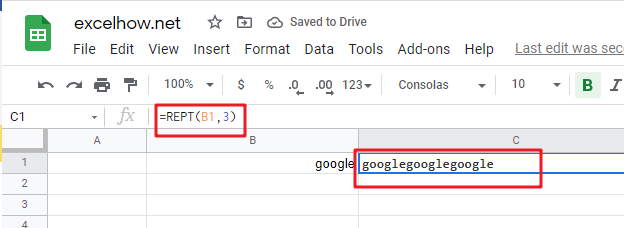#2 Repeat “g” letter 5 times, just using the following formula:

`=REPT(“g”,5) //it returns “ggggg”`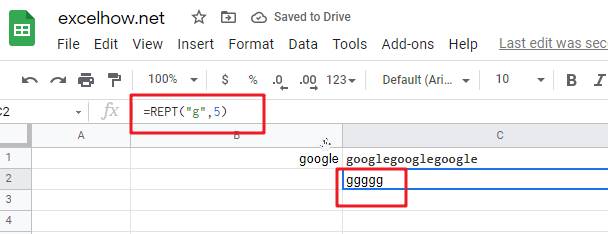#3 Repeat text string in Cell B1 using a specified number of times in Cell C1, you can type the following REPT formula in Cell D1:

`=REPT(B1,C1) //it returns “googlegooglegoogle”`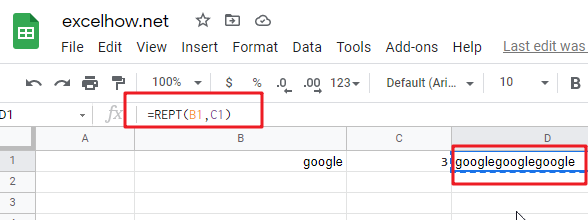This post will guide you how to use Google Sheets REPLACEB function with syntax and examples.

## Description

The Google Sheets REPLACEB function replaces all or part of a text string with another text string based on a number of bytes.

The REPLACEB function can be used to replace characters specified by position in the given text string with another newly text string in google sheets.

The REPLACEB function is a build-in function in Google Sheets and it is categorized as a Text Function.

## Syntax

The syntax of the REPLACEB function is as below:

=REPLACEB (old_text, start_num, num_bytes, new_text)

Where the REPLACEB function arguments are:

• Old_text -This is a required argument. The text string that you want to replace all or part of
• Start_num – This is a required argument. The position of the first character that you want to replace within old_text.
• Num_bytes – This is a required argument. The number of characters that you want to replace within old_text
• New_text – This is a required argument. The new text that will replace characters in old_text text string.

Note:

• The returned results of REPLACEB function are treated as text string in google sheets and if you are using the REPLACEB function with numbers in calculations, the error message may be returned.
• The REPLACEB function returns text as the output. And if you wish to return a number, and you can try to use the VALUE function in combination with the REPLACEB function.
• The REPLACEB function takes 4 required arguments. The first argument, `old_text`, is the text string that a part of which will be replaced. The second argument, `Start_num` is the position where the replacement will begin to replace. The third argument, `Num_bytes` is the number of bytes in the text to be replaced. The last argument, `New_text` is the text which will be inserted into the original text string.
• If both `Start_num` and `Num_bytes` are not a positive number and the REPLACEB function returns a #VALUE error.
• It is designed to work with double-type characters set. Such as: simplified Chinese.
• REPLACEB function will return the same value as REPLACE function if the `old_text` has only single byte characters.

## Google Sheets REPLACE Function Examples

The below examples will show you how to use google sheets REPLACEB function to replace part of a text string with another new text string.

#1 To replace 4 bytes in B1 cell with a new text string and starting with 7th character in old_text text, just using formula:

`=REPLACEB(B1,7,4," replace") //it returns “google replace1 sheets 2020”`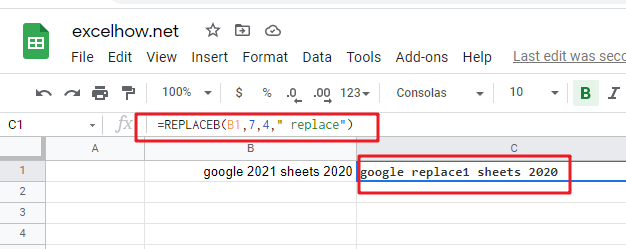Note: You can use the REPLACEB function to replace a newly text string at a given position in a text string. And you can use the SUBSTITUTE function to replace an existing text with a new text in a string. And Using FIND function and SEARCH function to get the location of a given text string in a text sting.